﻿ A Trapezoidal Fuzzy Method for Assessing Students’ Mathematical Modeling Skills

### A Trapezoidal Fuzzy Method for Assessing Students’ Mathematical Modeling Skills

Michael Gr. VoskoglouOPEN ACCESSPEER-REVIEWED

## A Trapezoidal Fuzzy Method for Assessing Students’ Mathematical Modeling Skills

Michael Gr. VoskoglouSchool of Technological Applications, Graduate Technological Educational Institute (T. E. I.) of Western Greece, Patras, Greece

### Abstract

Fuzzy logic due to its nature of characterizing each case with multiple values offers a rich field of resources covering the assessment of situations characterized by a degree of vagueness and/or uncertainty. In this paper, we apply a new trapezoidal fuzzy assessment model (TRFAM) for measuring students’ mathematical modeling (MM) skills. TRFAM is actually a variation of the commonly used in fuzzy logic centre of gravity (COG) defuzzification technique, which we have properly adapted and utilized in earlier papers as an assessment model. Our COG and TRFAM models focus on students’ quality performance by assigning greater coefficients to the higher scores. A classroom experiment is also presented, illustrating our results in practice. Through this experiment the above two models are compared to each other and also with two traditional assessment methods based on principles of bivalent logic (the calculation of means and of the GPA index) and some useful conclusions are drawn.

### At a glance: Figures

12
Prev Next

• Voskoglou, Michael Gr.. "A Trapezoidal Fuzzy Method for Assessing Students’ Mathematical Modeling Skills." American Journal of Educational Research 2.12 (2014): 1144-1150.
• Voskoglou, M. G. (2014). A Trapezoidal Fuzzy Method for Assessing Students’ Mathematical Modeling Skills. American Journal of Educational Research, 2(12), 1144-1150.
• Voskoglou, Michael Gr.. "A Trapezoidal Fuzzy Method for Assessing Students’ Mathematical Modeling Skills." American Journal of Educational Research 2, no. 12 (2014): 1144-1150.

 Import into BibTeX Import into EndNote Import into RefMan Import into RefWorks

### 1. Introduction

The assessment of a system’s effectiveness (i.e. of the degree of attainment of its targets) with respect to an activity performed within the system (e.g. problem-solving, decision making, learning process, etc) is a very important task that enables the correction of the system’s weaknesses resulting to the improvement of its general performance.

The assessment methods that are commonly used in practice are based on principles of the bivalent logic (yes-no). However, there are cases where a crisp characterization is not probably the proper one for an assessment. In the area of Education, for example, a teacher is frequently not sure about a particular numerical grade characterizing a student’s performance. Fuzzy logic, due to its nature of characterizing a case with multiple values, offers wider and richer resources for covering such kind of cases than the bivalent logic does.

The several methods being in use for assessing a system’s performance focus on different targets: Some of them measure the mean system’s performance, while others measure its quality performance by assigning greater coefficients (weights) to the higher scores. Therefore, one who wants to obtain a comprehensive view of a system’s performance should make use of more than one assessment methods for this purpose.

In this paper we apply a new fuzzy model for assessing students’ mathematical modelling (MM) skills. The rest of the paper is organized as follows: In section 2 we attempt a brief description of the MM process (circle of modelling) as a method for teaching mathematics. In section 3 we develop our new trapezoidal fuzzy assessment model (TRFAM), which is actually a variation of the centre of gravity (COG) defuzzification technique that we have properly adapted and used in earlier papers as an assessment method. An application on MM is presented in section 4 illustrating our results in practice. Through this application the COG and TRAMF methods are compared to each other and with other traditional assessment methods (calculation of the means and of the GPA index). We close by stating, in section 5, our final conclusions and our future plans for further research on the subject.

For general facts on fuzzy sets we refer to the book .

### 2. The circle of Modelling

Until the middle of 1970’s mathematical modelling (MM) was mainly a tool in hands of scientists and engineers for solving real world problems related to their disciplines (physics, industry, constructions, economics, etc). One of the first who described the process of MM in such a way that it could be used for teaching mathematics was Pollack . He represented the interaction between mathematics and the real world with the scheme shown in Figure 1, which is known as the circle of modelling.

According to the above scheme, in the “universe” of mathematics classical applied mathematics and applicable mathematics are two intersected but not equal to each other sets. In fact, there are topics from classical mathematics with great theoretical interest, but without any visible applications (although such applications it is possible to be found in future), while at the same time they are branches of mathematics with many practical applications, which are not characterized by the experts as classical mathematics (e.g. statistics, fuzzy logic, fractals, etc). But the most important feature in Pollack’s scheme is the direction of the arrows, representing a looping between the other (real) world, (including all the other sciences and the human activities of everyday life) and the “universe” of mathematics: Starting from a problem of the real world we transfer to the other part of the scheme, where we use or develop suitable mathematics for its solution. Then we return to the real world interpreting and testing the mathematical results obtained. If these results are not giving a satisfactory solution to the problem, then we repeat the same circle again one or more times.

From the time that Pollack presented this scheme in ICME-3 (Karlsruhe, 1976) until nowadays much effort has been placed to analyze in detail the process of MM as a method of teaching mathematics (Barry & Davies , Edwards & Hauson , Blomhψj & Jensen , Greefrath , Blum & Leib , Voskoglou , etc). A brief but comprehensive account of the different models used for the description of the MM process can be found in Chaines & Crouch  including our stochastic model [[16, 17, 18] (section 5.2)].

As a result of all these research efforts it is more or less acceptable nowadays that the process of MM basically involves the following stages:

S1: Analysis of the problem (understanding the statement and recognizing the restrictions and requirements of the real system.

S2: Mathematization (formulation of the problem and construction of the model).

S3: Solution of the model.

S4: Validation (control) of the model, which is achieved by reproducing, through the model, the behavior of the real system under the conditions existing before the construction of the model. In case of a system having no previous “history” an extra (usually simulation) model can be used for this purpose.

S5: Interpretation of the final mathematical results and implementation of them to the real system, in order to give the “answer” to the real world problem.

In  we have treated the MM circle as a Markov chain process dependent upon the transition between the discrete stages of the MM process. The arrows in Figure 2 are showing the possible transitions between stages.

Some authors consider further stages in the MM process; e.g. some of them divide mathematization to the stages of formulation of the real problem in a way that it will be ready for mathematical treatment and of construction of the model, others divide the validation to the stages of interpretation and evaluation of the model, others add the stage of refining the model, etc (e.g. see ). However, all these minor variations do not change the general idea that we nowadays have about the circle of MM.

MM appears today as a dynamic tool for teaching and learning mathematics, because it connects mathematics with our everyday life giving the possibility to students to understand its usefulness in practice and therefore increasing their interest about mathematics. In other words, according to the Polya’s  terminology (axioms of learning), MM works as a best motivation for learning mathematics. But we must be careful! The process of MM could not be considered as a general and therefore applicable in all cases method for teaching mathematics. In fact, such a consideration could lead to far-fetched situations, where more emphasis is given to the search of the proper application rather, than to the consolidation of the new mathematical knowledge!

### 3. Development of the TRFAM

The COG (or centroid) technique is a commonly used in fuzzy logic defuzzification method (e.g. see ). In earlier papers (e.g. [13, 20, 21], etc) the COG technique has been properly adapted to be used as an assessment method.

In fact, let G be a group of individuals participating in a certain activity and let U={A, B, C, D, F} be a set of linguistic labels (grades) characterizing the individuals’ performance with respect to this activity as follows: A=excellent, B=very good, C=good, D=moderate (satisfactory) and F=unsatisfactory. Then, we can express G as a fuzzy set in U in the form G = {(x, m(x)), x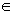U}, where y=m(x) is the corresponding membership function.

In this case, according to our results (e.g. see section 2 of ) the group’s performance can be measured by the coordinates (xc, yc) of the COG of the level’s section contained between the graph of the membership function y=m(x) (which in our case turns out to be a bar graph consisting of five rectangles; e.g. see Figure 1 of ) and the OX axis, which are calculated by the formulas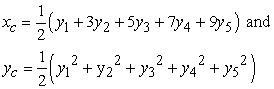(1)

with y1=m(F), y2=m(D), y3 =m(C), y4 =m(B), y5 =m(A) and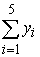= 1 (the values of the yi’s can be normalized by dividing each of them by their sum). The ideal case appears when all the individuals of the group demonstrate excellent performance, i.e. when y1=y2=y3=y4=0 and y5=1. In this case formulas (1) give that the COG is the point (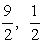).

Further, the following criterion holds for comparing the performance of two (or more) groups:

Between two groups, the group with the greater value of xc performs better. Also, if the value of xc is equal for both groups, then, if xc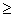2.5, the group with the greater value of yc performs better, while, if xc < 2.5, then the group with the smaller value of yc performs better.

The TRFAM is a recently developed variation of the COG method . The important novelty of this approach is in the replacement of the rectangles appearing in the graph of the membership function of the COG method by isosceles trapezoids sharing common parts. In the TRFAM’s scheme (Figure 3) we have five trapezoids, corresponding to the above defined grades F, D, C, B and A respectively of the individuals’ performance. Without loss of generality and for making our calculations easier we consider isosceles trapezoids with bases of length 10 units lying on the OX axis. The height of each trapezoid is equal to the percentage of individuals who achieved the corresponding grade for their performance, while the parallel to its base side is equal to 4 units. We allow for any two adjacent trapezoids to have 30% of their bases (3 units) belonging to both of them. In this way we cover the ambiguous cases of individuals’ scores being at the boundaries between two successive grades. For students’ assessment, for example, it is a very common approach to divide the interval of the specific grades in three parts and to assign the corresponding grade using + and -. For example, 75 – 77% = B-, 78 – 81% = B, 82 – 84% = B+. However, this consideration does not reflect the common situation, where the teacher is not sure about the grading of the students whose performance could be assessed as marginal between and close to two adjacent grades; for example, something like 84 - 85 being between B+ and A-. The TRFAM fits this situation.

An individuals’’ group can be represented, as in the COG method, as a fuzzy set in U, whose membership function y=m(x) has as graph the line OB1C1H1B2C2H2B3C3H3B4C4H4B5C5D5 of Figure 2, which is the union of the line segments OB1, B1C1, C1H1,.., B5C5, C5D5. However, in case of the TRFAM the analytic form of y = m(x) is not needed for calculating the COG of the resulting area. In fact, since the boundary cases of the individuals’ scores are considered as common parts for any pair of the adjacent trapezoids, it is logical to count these parts twice; e.g. placing the ambiguous cases B+ and A- in both regions B and A. In other words, the COG method, which uses the analytic form of y = m(x) for calculating the coordinates of the COG of the area between the graph of the membership function and the OX axis, thus considering the areas of the “common” triangles A2H1D1, A3H2D2, A4H3D3 and A5H4D4 only once, is not the proper one to be applied in the above situation.

Therefore, in this case we represent each one of the five trapezoids of Figure 3 by its COG Fi, i=1, 2, 3, 4, 5 and we consider the entire area, i.e. the sum of the areas of the five trapezoids, as the system of these points-centers. More explicitly, the steps of the whole construction of the TRFAM are the following:

1. Let yi, i=1, 2, 3, 4, 5 be the percentages of the individuals’ whose performance was characterized by F, D, C, B, and A respectively; then=1 (100%).

2. We consider the isosceles trapezoids with heights equal to yi, i=1, 2, 3, 4, 5, in the way that has been illustrated in Figure 2.

3. We calculate the coordinates (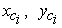) of the COG Fi, i=1, 2, 3, 4, 5, of each trapezoid as follows: It is well known that the COG of a trapezoid lies along the line segment joining the midpoints of its parallel sides a and b at a distance d from the longer side b given by d=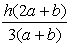, where h is its height (e.g. see ). Therefore in our case we have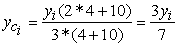Also, since the abscissa of the COG of each trapezoid is equal to the abscissa of the midpoint of its base, it is easy to observe that xci=7i-2.

4. We consider the system of the COG’s Fi, i=1, 2, 3, 4, 5 and we calculate the coordinates (Xc, Yc) of the COG Fc of the whole area S considered in Figure 2 by the following formulas, derived from the commonly used in such cases definition (e.g. see ):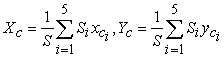(2)

In formulas (2) Si, i= 1, 2, 3, 4, 5 denote the areas of the corresponding trapezoids. Thus, Si=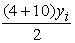=7yi and S =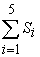= 7= 7. Therefore, from formulas (2) we finally get that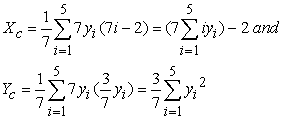(3)

5. We determine the area where the COG Fc lies as follows: For i, j=1, 2, 3, 4, 5, we have that 0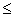(yi -yj)2=yi2+yj2-2yiyj, therefore yi2+yj22yiyj, with the equality holding if, and only if, yi=yj. Thus,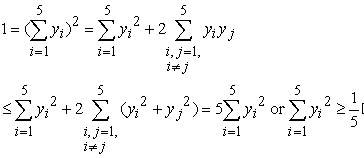(4)

with the equality holding if and only if y1 = y2 = y3 = y4 = y5 =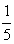. In the case of equality the first of formulas (3) gives that Xc = 7(+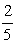+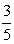+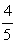+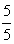) – 2 = 15. Further, combining the inequality (4) with the second of formulas (3) one finds that Yc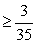Therefore the unique minimum for Yc corresponds to the COG Fm(15,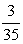). The ideal case is when y1=y2=y3= y4=0 and y5=1. Then from formulas (3) we get that Xc = 33 and Yc =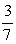.Therefore the COG in this case is the point Fi (33,). On the other hand, the worst case is when y1=1 and y2= y3 = y4= y5=0. Then from formulas (3), we find that the COG is the point Fw(5,). Therefore the area where the COG Fc lies is the area of the triangle Fw Fm Fi (see Figure 4).

6. We formulate our criterion for comparing the performances of two (or more) different groups’ as follows: From elementary geometric observations (see Figure 3) it follows that for two groups the group having the greater Xc performs better. Further, if the two groups have the same Xc ≥15, then the group having the COG which is situated closer to Fi is the group with the greater Yc. Also, if the two groups have the same Xc<15, then the group having the COG which is situated farther to Fw is the group with the smaller Yc. Based on the above considerations we obtain the following criterion:

•  Between two groups the group with the greater value of Xc demonstrates the better performance.

•  If two groups have the same Xc 15, then the group with the greater value of Yc demonstrates the better performance.

•  If two groups have the same Xc < 15, then the group with the smaller value of Yc demonstrates the better performance.

Once developed, the TRFAM is very easy to be applied in practice, because it needs no complicated calculations in its final step. The same also holds for the COG technique.

Observing the first of formulas (1) and (3) we can see that both of the above fuzzy assessment methods assign for the abscissa of the COG greater coefficients to the higher scores. Therefore, since the value of the COG’s abscissa measures in both cases the group’s performance (see the above criterion), we conclude that these fuzzy assessment methods focus on the quality performance rather than on the mean performance of the corresponding group.

### 4. An Application to MM

Exploratory investigations have demonstrated how exposure to computers enhances the way students approach MM problems (Asiala et al. , Weller et al. , Lewandowski et al. , Yadav et al. , Voskoglou & Buckley , etc).

In our will to explore further the effect of the use of computers as a tool for solving MM problems we performed the following classroom experiment with subjects students of the School of Technological Applications (prospective engineers) of the Graduate Technological Educational Institute (T. E. I.) of Western Greece attending the course “Higher Mathematics I” of their first term of studies, which involves Differential and Integral Calculus in one variable, Elementary Differential Equations and Linear Algebra. The students, who had no previous experience on computers apart from the basics learned in secondary education, were divided in two equivalent groups according to their grades obtained in the Panhellenic maths exam for entrance in higher education.

For the control group the lectures were performed in the classical way on the board, followed by a number of exercises and examples connecting mathematics with real world applications and problems. The students participated in solving these problems. The difference for the experimental group was that about the 1/3 of the lectures and exercises were performed in a computer laboratory. There the instructor using the computers presented the corresponding mathematical topics in a more “live” and attractive way, while the students themselves, divided in small groups and making use of known mathematical software packages solved the problems with the help of computers. Notice that the teaching schedule of the course involved six hours per week for both groups including the time spent in the computer laboratory for the experimental group.

At the end of the term all students participated to the final written exam of the course for the assessment of their progress. The exam involved a number of general theoretical questions and exercises covering all the topics taught and three simplified real world problems (see Appendix) requiring MM techniques for their solutions. We marked the students’ papers in a scale from 0 to 100, separately for the questions and exercises and separately for the problems. Further, we characterized their performance as follows: A (90-100) = Excellent, B (75-89) = Very Good, C (60-74) = Good, D (50-59) = Satisfactory and E (0-49) = Unsatisfactory.

No significant differences were found between the two groups concerning the theoretical questions and exercises, their overall performances were almost identical. Below we shall present and evaluate in detail the results of the two groups concerning the MM problems. The scores achieved by the students of the two groups were the following:

Experimental group (G1): 100(5 times), 99(3), 98(10), 95(15), 94(12), 93(1), 92 (8), 90(6), 89(3), 88(7), 85(13), 82(4), 80(6), 79(1), 78(1), 76(2), 75(3), 74(3), 73(1), 72(5), 70(4), 68(2), 63(2), 60(3), 59(5), 58(1), 57(2), 56(3), 55(4), 54(2), 53(1), 52(2), 51(2), 50(8), 48(7), 45(8), 42(1), 40(3), 35(1).

Control group (G2) : 100(7), 99(2), 98(3), 97(9), 95(18), 92(11), 91(4), 90(6), 88(12), 85(36), 82(8), 80(19), 78(9), 75(6), 70(17), 64(12), 60(16), 58(19), 56(3), 55(6), 50(17), 45(9), 40(6).

The above data is summarized in Table 1:

#### Table 1. Characterization of the students’ performance

The evaluation of the above data was performed in two ways:

a) Calculation of the means: A straightforward calculation gives that the means of the students’ scores are approximately 76.006 and 75.09 for the experimental and the control group respectively. This shows that the mean performances of both groups were very good (on the boundary), with the performance of the experimental group being slightly better.

b) Calculation of the Great Point Average (GPA) index: We recall that the GPA index is a weighted mean, focusing on the quality performance of a student group (e.g. see ). Let us denote by nA, nB, nC, nD and nE the numbers of students whose performance was characterized by A, B, C, D and F respectively and by n the total number of students of each group.. Then the GPA index is calculated by the formula GPA=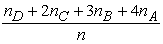. Obviously we have that

0GPA4 (in the worst case n=nF, while in the ideal case n=nA). In terms of the terminology introduced in the TRFAM (see paragraph 1 of section 3) the above formula can be written in the form: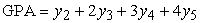(5)

In our case, applying formula (5) on the data of Table 1 we find that the GPA of both student groups’ is equal to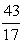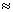2.53. This is a satisfactory value for the GPA index, since it is equal to the 63.25% of its maximal possible value, which is equal to 4. Thus, according to the GPA index, the two student groups demonstrated the same (good) quality performance.

II) Fuzzy logic methods

c) The COG method: Applying the first of formulas (3) on the data of Table 1 one finds that the coordinate xc of the COG for both G1 and G2 is equal to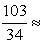3.03 > 2.5. Thus, since the value 3.03 is approximately equal to the 67.3% of the corresponding value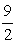of the ideal case, both groups demonstrated a good quality performance. Further, from the second of formulas (3) it turns out that the coordinate yc is equal to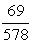for G1 and to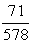for G2. Therefore, according to our criterion for the COG technique stated in section 3, the control group demonstrated a slightly better performance.

d) Application of the TRFAM: Applying the data of Table 1 on the first of formulas (5) one finds that the coordinate Xc of the whole area S considered in Figure 2 is equal to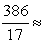22.71>15 for both groups. The value 22.71 is approximately equal to the 66.67% of the corresponding value of the ideal case, which shows again that both groups demonstrated a good quality performance. Further, from the second of formulas (3) we find that that the coordinate yc is approximately equal to 0.102 for G1 and to 0.107 for G2. Therefore, according to our criterion for the TRFAM stated in section 3, the control group demonstrated a slightly better performance.

III) Discussion of the experimental results

The application of the above - four in total - methods for assessing students’ MM skills resulted to different conclusions. However, this is not embarrassing, since, in contrast to the calculation of the means which focuses on the mean performance of a student group, the GPA the COG and the TRFAM methods focus on its quality performance by assigning weight coefficients to the higher scores achieved by students.

The coefficients attached to the yi’s in the last three methods -see formula (5) and the first of formulas (1) and (3) respectively- are presented in Table 2. From this Table becomes evident that TRFAM assigns greater coefficients to the higher with respect to the lower scores than COG and also COG does the same thing with respect to GPA. In other words TRFAM is more sensitive than COG, and COG is more sensitive than GPA for measuring the quality performance of a student group.

#### Table 2. Weight coefficients of the yi’s

Concerning the effect of the use of computers for enhancing students MM skills that we wanted to investigate with the above experiment, according to the results obtained it looks to improve the mean performance of the experimental with respect to the control group. On the contrary the GPA index shows that the quality performance of the two groups was the same, while the GOC and TRFAM methods show a slight superiority of the control group. In other words, the use of computers enhanced the performance of the moderate students (lower scores), but it had no effect on the performance of the good students (higher scores). An explanation about this could be that the figures’ animation, the quick transformations of the numerical and algebraic representations, the easy and accurate construction of the several graphs especially in the 3-dimensional space, etc, which are comfortably achieved using the computers, increased the moderate students’ imagination and helped them in using their intuition more effectively for designing the solutions of the corresponding problems. On the contrary, this had no effect on the good students, who had already developed high MM skills.

### 5. Final Conclusions and Discussion

In the present paper methods were developed and/or applied for assessing students’ model building abilities with emphasis to our new TRFAM model. A classroom experiment, performed recently at the Graduate T. E. I. of Western Greece, was also presented illustrating our results in practice and connecting students’ MM skills with the use of computers.

According to the results of the above experiment small differences appeared between the performances of the experimental and the control group giving a rather weak indication about the positive effect of computers for enhancing students’ MM skills.. This, combined with the results of older similar researches mentioned in section 4, underlines the need of further experimental investigation for obtaining safer statistical conclusions.

Further, the TRAFM seems to have the potential of a general assessment method that could have many other applications in future on several sectors of human activity (not on Education only) and this is another priority of our future research plans on this topic.

### References

  Asiala, M., et al., “A framework for research and curriculum development in undergraduate mathematics education”, Research in Collegiate Mathematics Education II, CBMS Issues in Mathematics Education, 6, 1-32, 1996.In article  Berry, J. and Davies, A., “Written reports, Mathematics Learning and Assessment: Sharing Innovative Practices”, in Haines, C. R. & Dunthornr (Eds.), London, Arnold, 1996.In article  Blomhψj, M. and Jensen, T. H., “Developing mathematical modelling competence: Conceptual clarification and educational planning”, Teaching Mathematics and its Applications, 22, 123-129, 2003.In article CrossRef  Blum, W. & Leii, “How do students and teachers deal with modelling problems?’. In C. Chaines, P. Galbraith, W. Blum & S. Khan (Eds.): Mathematical Modelling (ICTMA 12): Education, Engineering and Economics, Chichester, Horwood Publishing, 222-231, 2007.In article  Edwards D. & Hamson M. J., Mathematical Modelling Skills, London, Macmillan, 1996.In article  “Grade Point Average Assessment”, available in the Web at: http://www.swinburne.edu.au/student-administration/assessment/gpa.html, visited October 15, 2014.In article  Greefrath, G., Modellieren lernen mitoffenen realitatsnahen Aufgaben, Koln, Aulis Verlag, 2007.In article  Haines, C. & Crouch, R., “Remarks on a Modelling Cycle and Interpretation of Behaviours’. In R.A. Lesh et al. (Eds.): Modelling Students’ Mathematical Modelling Competencies (ICTMA 13), 145-154, Springer, US, 2010In article  Klir, G. J. & Folger, T. A.., Fuzzy Sets, Uncertainty and Information, Prentice-Hall, London, 1988.In article  Lewandowski, G. et al., “Common sense computing (episode 3): Concurrency and concert tickets”, Proceedings of the Third International Workshop on Computing Education Research (ICER), 2007.In article CrossRef  Pollack H. O., “The interaction between Mathematics and other school subjects”, New Trends in Mathematics Teaching, Volume IV, Paris: UNESKO, 1979.In article  Polya, G., “On learning, teaching and learning teaching”, American Mathematical Monthly, 70, 605-619, 1963.In article CrossRef  Subbotin, I. Ya., Badkoobehi, H., Bilotckii, N. N., “Application of fuzzy logic to learning assessment”. Didactics of Mathematics: Problems and Investigations, 22, 38-41, 2004.In article  Subbotin, I. Ya., Voskoglou, M. Gr., “Fuzzy Models for Learning Assessment”, arXiv: 1410.4497 [math. OC], submitted on October 15, 2014.In article  van Broekhoven, E. & De Baets, B., “Fast and accurate centre of gravity defuzzification of fuzzy system outputs defined on trapezoidal fuzzy partitions”, Fuzzy Sets and Systems, 157 (7), 904-918.In article CrossRef  Voskoglou, M. Gr. (1994), An application of Markov Chain to the process of modelling, International Journal of Mathematical Education in Science and Technology, 25 (4), 475-480, 2006.In article CrossRef  Voskoglou, M. Gr., “A stochastic model for the modelling process”. In C. Chaines, P. Galbraith, W. Blum & S. Khan (Eds.): Mathematical Modelling (ICTMA 12): Education, Engineering and Economics, Chichester, Horwood Publishing, 149-157, 2007.In article  Voskoglou, M. Gr., Stochastic and fuzzy models in Mathematics Education, Artificial Intelligence and Management, Lambert Academic Publishing, Saarbrucken, Germany, 2011. For more details look at http://amzn.com./3846528218).In article  Voskoglou, M. Gr. & Buckley, S., “Problem Solving and Computers in a Learning Environment”, Egyptian Computer Science Journal, 36 (4), 28-46, 2012.In article  Voskoglou, M. Gr. & Subbotin, I. Ya., “Dealing with the Fuzziness of Human Reasoning”, International Journal of Applications of Fuzzy Sets and Artificial Intelligence, Vol. 3, 91-106, 2013.In article  Voskoglou, M. Gr., “Assessing the Players’ Performance in the Game of Bridge: A Fuzzy Logic Approach, American Journal of Applied Mathematics and Statistics, 2 (3), 115-120, 2014.In article CrossRef  Weller, K. et al., “Student performance and attitudes in courses based on APOS theory and the ACE teaching style”. In A. Selden et al. (Eds.), Research in collegiate mathematics education V, 97- 13, Providence, RI, American Mathematical Society, 2003.In article  Wikipedia, “Trapezoid: Other properties”, available in the Web at: http://en.wikipedia.org/wiki/trapezoid#other_properties, visited on October 10, 2014.In article  Wikipedia, “Center of mass: A system of particles”, available in the Web at: http://en.wikipedia.org/wiki/Center_of_mass#A_system_of_particles, visited on October 10, 2014.In article  Yadav, A. et al., “Introducing Computational Thinking in Education Courses”, Proceedings of the 42nd ACM technical symposium on Computer Science Education 11, 465-470, March 9-12, 2011.In article PubMed

### APPENDIX: List of MM problems given for solution in our experiment

Problem 1: Among all the cylindrical towers having a total surface of 180π m2, which one has the maximal volume?

Problem 2: Let us correspond to each letter the number showing its order into the alphabet (A=1, B=2, C=3 etc). Let us correspond also to each word consisting of 4 letters a 2X2 matrix in the obvious way; e.g. the matrix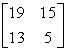corresponds to the word SOME. Using the matrix E=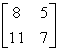as an encoding matrix how you could send the message LATE in the form of a camouflaged matrix to a receiver knowing the above process and how he (she) could decode your message?

Problem 3: The population of a country is increased proportionally. If the population is doubled in 50 years, in how many years it will be tripled?